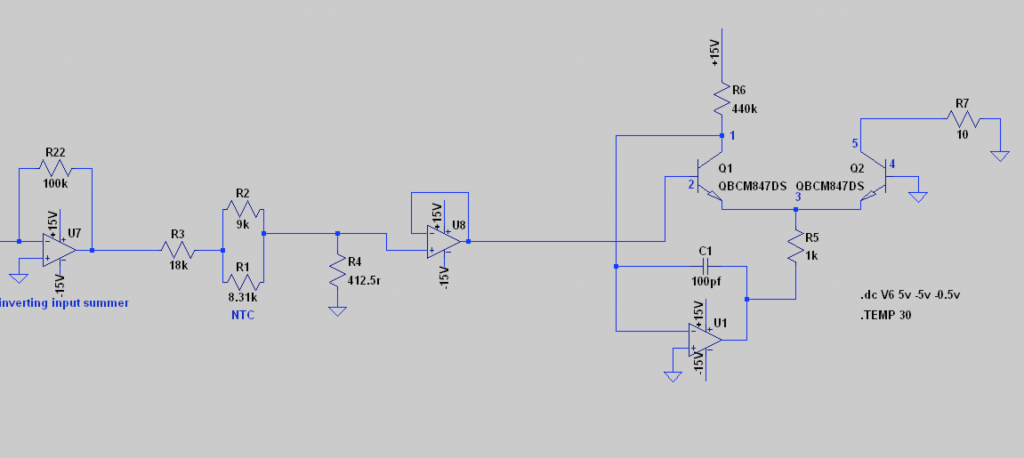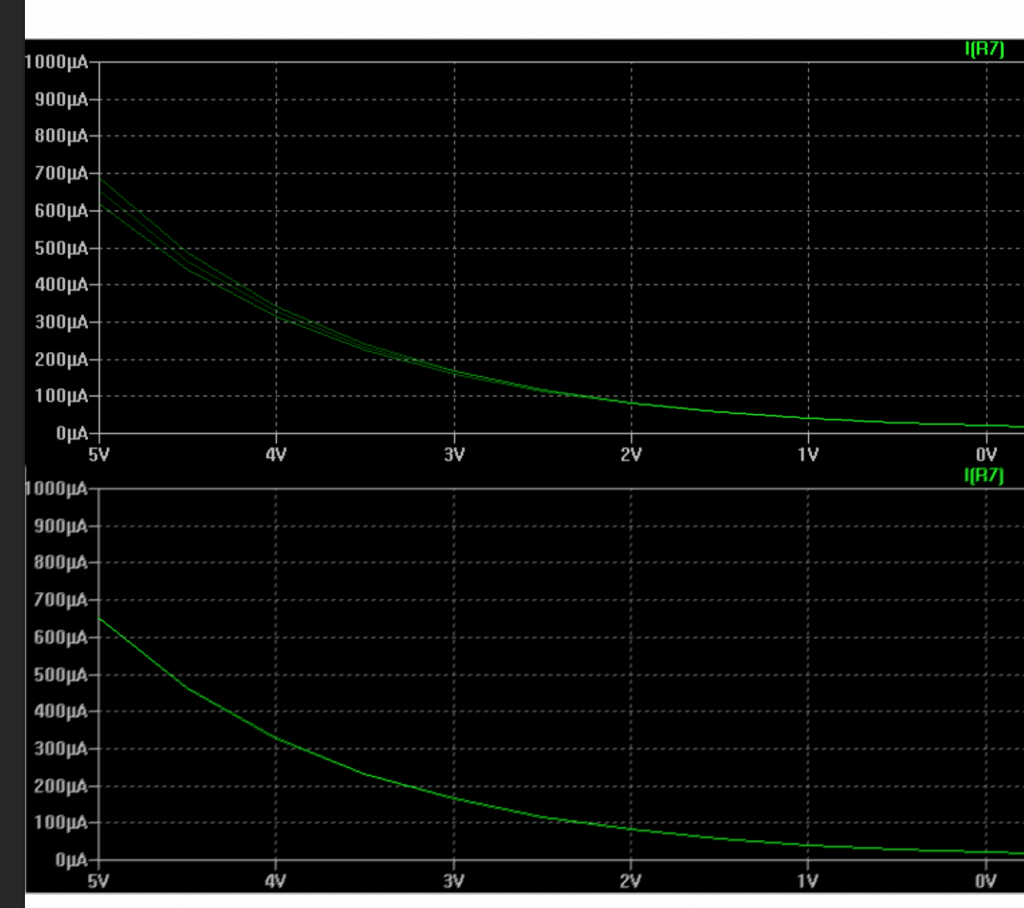# a modern take on the exponential converter

In the previous post, I investigated the possibility to replace the CA3046 and expo pair with modern components in a synthesizers’ exponential converter, while retaining the temperature compensation. Continuing on the findings from the previous post, I worked out a schematic:CV inputs are summed in opamp U7, then scaled with regard to temperature over R1 through R4 for the expo converter build up out of a BCM847DS matched transistor pair. R1 is a specific SMD NTC thermistor. The value of R2 is calculated via a linearization formula I found on the internet. The actual value for linearization was something of 7.5k, but an Excel sheet approximation showed that 9k would work better in normal room temperatures (15 – 25 degrees). R4 determines the to-ground part of the voltage divider (with R1-R2-R3) and thus sets the 1V per octave setting and should be variable for a small part.

Simulation of 3 temperatures shows the following:The top plot shows the difference in ua output over R7 for the three temperatures (20, 25 and 30 degrees Celcius). The bottom plots shows the outputs for the temperatures over R7, but this time compensated by the thermistor circuit. Yes, no difference!

Now on to building my first commercial Eurorack component. A triple VCO under the bennis|budge brand. Keep watching this space ;)